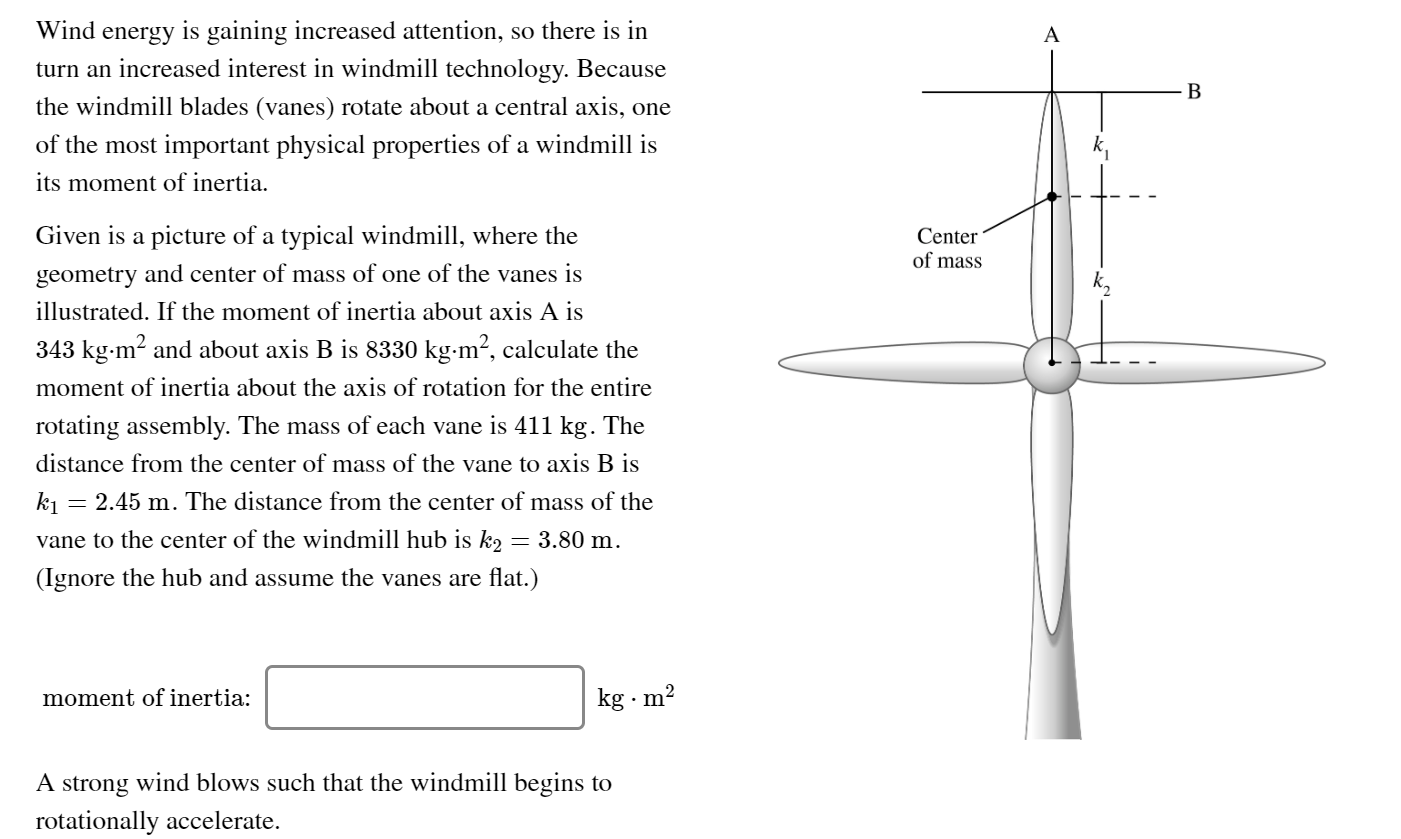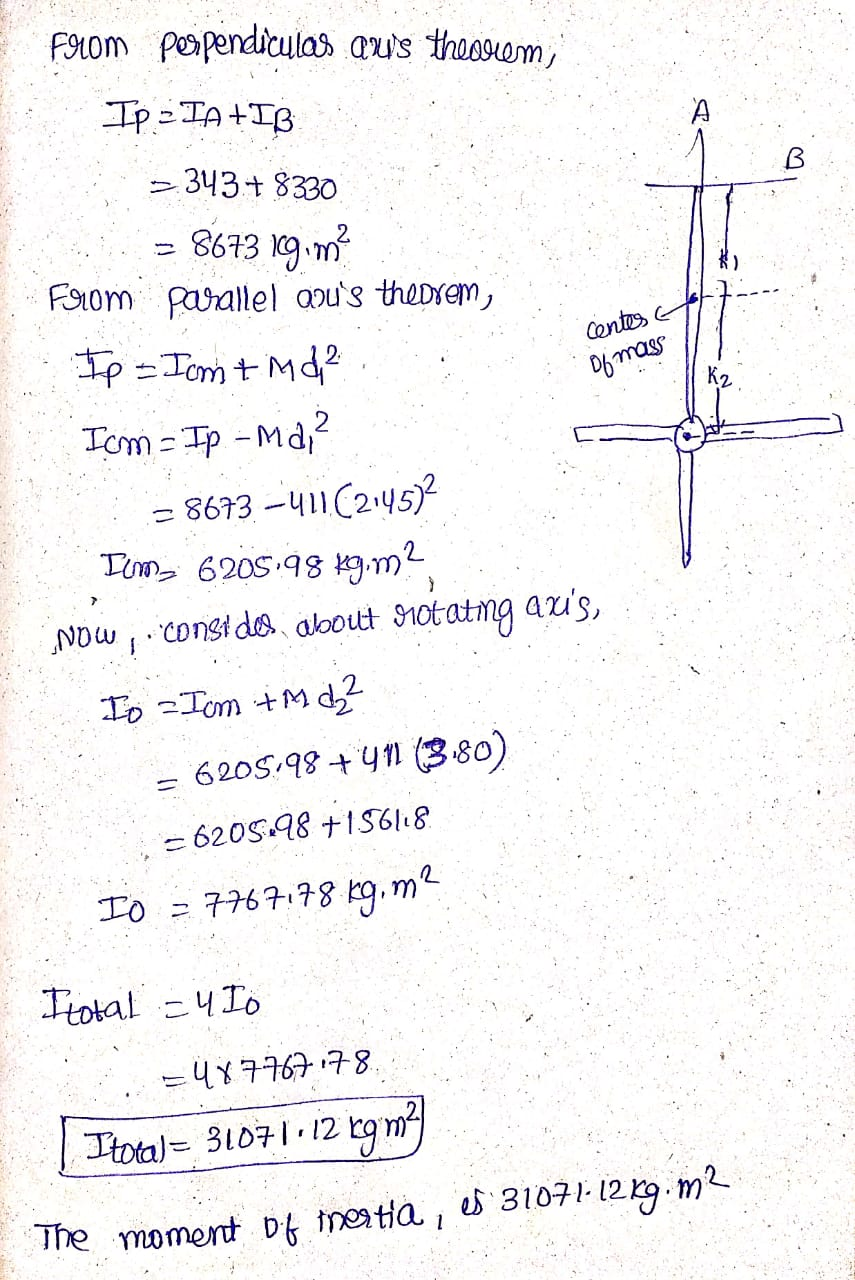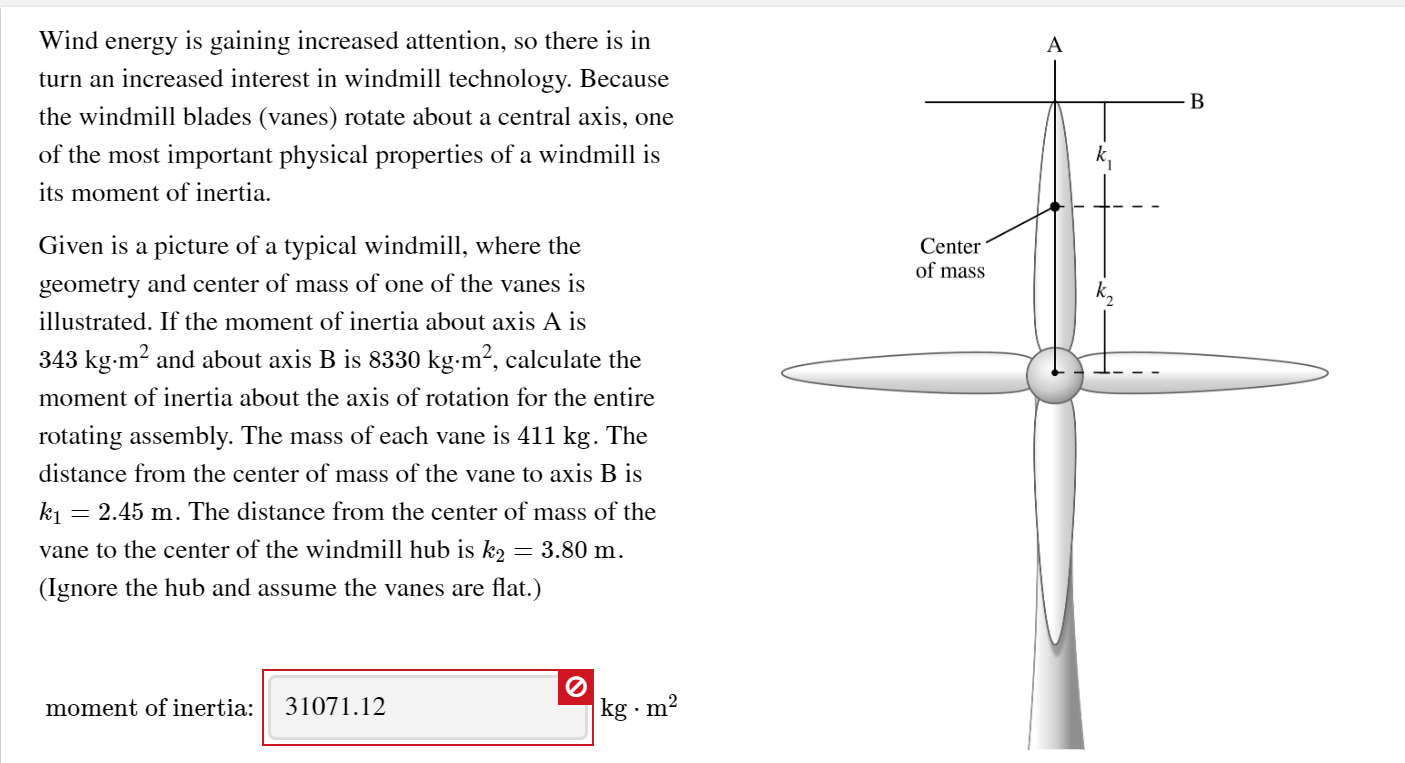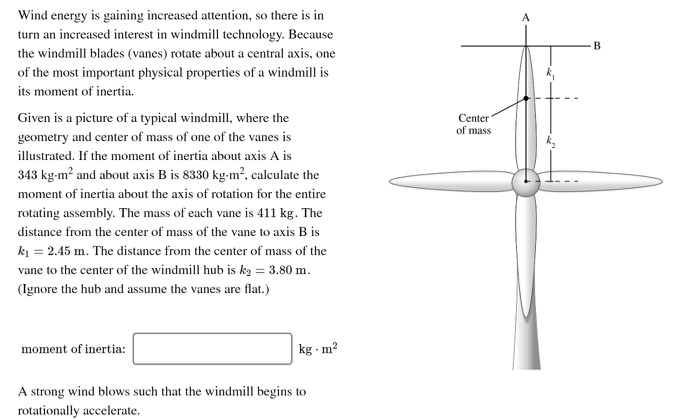# Wind energy is gaining increased attention, so there is in turn an increased interest in windmill...Wind energy is gaining increased attention, so there is in turn an increased interest in windmill technology. Because the windmill blades (vanes) rotate about a central axis, one of the most important physical properties of a windmill is its moment of inertia. Center of mass Given is a picture of a typical windmill, where the geometry and center of mass of one of the vanes is illustrated. If the moment of inertia about axis A is 343 kg.m2 and about axis B is 8330 kg.m, calculate the moment of inertia about the axis of rotation for the entire rotating assembly. The mass of each vane is 411 kg. The distance from the center of mass of the vane to axis B is kı = 2.45 m. The distance from the center of mass of the vane to the center of the windmill hub is k2 = 3.80 m. (Ignore the hub and assume the vanes are flat.) moment of inertia: kg · m2 A strong wind blows such that the windmill begins to rotationally accelerate.#### Earn Coin

Coins can be redeemed for fabulous gifts.

Similar Homework Help Questions
• ### Wind energy is gaining increased attention, so there is in turn an increased interest in windmill...Wind energy is gaining increased attention, so there is in turn an increased interest in windmill technology. Because the windmill blades (vanes) rotate about a central axis, one of the most important physical properties of a windmill is its moment of inertia. Center of mass - - - - Given is a picture of a typical windmill, where the geometry and center of mass of one of the vanes is illustrated. If the moment of inertia about axis A is...

• ### Center of mass Wind energy is gaining increased attention, so there is in turn an increased...Center of mass Wind energy is gaining increased attention, so there is in turn an increased interest in windmill technology. Because the windmill blades (vanes) rotate about a central axis, one of the most important physical properties of a windmill is its moment of inertia. Given is a picture of a typical windmill, where the geometry and center of mass of one of the vanes is illustrated. If the moment of inertia about axis A is 343 kg.mand about axis...